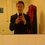# Fibonacci Investigation

A couple of months ago, we were studying graphs in Further Maths (not an ${xy}$ plane, rather a series of nodes and edges), when we decided to do a little investigation with ${tree}$ ${diagrams}$. The question goes like this: what are number of ${distinct}$ ${tree}$ ${diagrams}$ with ${n}$ nodes? Well we found that if a graph has (2,3,4,5,6,7) nodes then it would have (1,1,2,3,5,8) distinct tree diagrams respectively, which follows the Fibonacci sequence. We were not able to prove why, so could anybody be able to provide a proof? (I have a feeling it might be to do with the number of distinct ways you can write 2[${n}$-1] as a sum of ${n}$ numbers, if ${n}$ = number of nodes)Note by Curtis Clement
6 years, 7 months ago

This discussion board is a place to discuss our Daily Challenges and the math and science related to those challenges. Explanations are more than just a solution — they should explain the steps and thinking strategies that you used to obtain the solution. Comments should further the discussion of math and science.

When posting on Brilliant:

• Use the emojis to react to an explanation, whether you're congratulating a job well done , or just really confused .
• Ask specific questions about the challenge or the steps in somebody's explanation. Well-posed questions can add a lot to the discussion, but posting "I don't understand!" doesn't help anyone.
• Try to contribute something new to the discussion, whether it is an extension, generalization or other idea related to the challenge.

MarkdownAppears as
*italics* or _italics_ italics
**bold** or __bold__ bold
- bulleted- list
• bulleted
• list
1. numbered2. list
1. numbered
2. list
Note: you must add a full line of space before and after lists for them to show up correctly
paragraph 1paragraph 2

paragraph 1

paragraph 2

[example link](https://brilliant.org)example link
> This is a quote
This is a quote
    # I indented these lines
# 4 spaces, and now they show
# up as a code block.

print "hello world"
# I indented these lines
# 4 spaces, and now they show
# up as a code block.

print "hello world"
MathAppears as
Remember to wrap math in $$ ... $$ or $ ... $ to ensure proper formatting.
2 \times 3 $2 \times 3$
2^{34} $2^{34}$
a_{i-1} $a_{i-1}$
\frac{2}{3} $\frac{2}{3}$
\sqrt{2} $\sqrt{2}$
\sum_{i=1}^3 $\sum_{i=1}^3$
\sin \theta $\sin \theta$
\boxed{123} $\boxed{123}$

Sort by:

Can you define more precisely what you mean by "tree diagrams" ? It is known that (Cayley's formula) number of distinct labelled trees on $n$ vertices is $n^{n-2}$.

- 6 years, 7 months ago

A series of nodes and edges such that the graph is connected and there are no cycles. Also, the nodes have to be connected using the least number of nodes. Examples: (order of each node) 2 nodes = {1,1} 3nodes = {2,1,1} 4 nodes = {1,2,2,1} and {3,1,1,1} 5 nodes = (4,1,1,1,1}, {3,2,1,1,1} and {2,2,2,1,1} etc

- 6 years, 7 months ago

I count 6 trees with 6 nodes up to graph isomorphism.

- 6 years, 7 months ago

Check the order of your nodes - we didn't find any counter-examples as a class.

- 6 years, 7 months ago

OEIS A000055 agrees as well, the sequence proceed as 1, 1, 2, 3, 6, 11, 23, 47,...

- 6 years, 7 months ago

What is OEIS A000055 ?

- 6 years, 7 months ago

https://oeis.org/A000055

- 6 years, 7 months ago